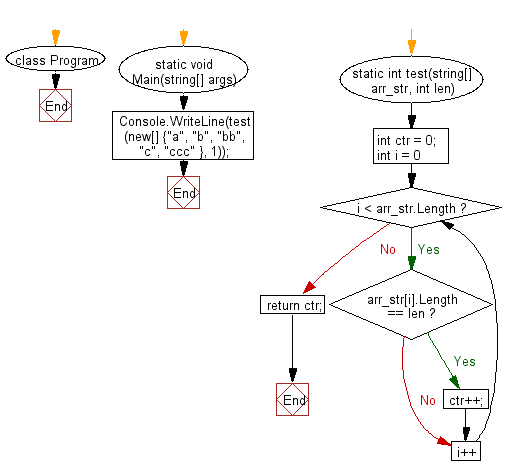﻿ C# - Count number of strings of a given length in an array# C# Sharp Basic Algorithm Exercises: Count the number of strings of specified length in a given array of strings

## C# Sharp Basic Algorithm: Exercise-136 with Solution

Write a C# Sharp program to count the number of strings of specified length in a given array of strings.

Sample Solution:

C# Sharp Code:

``````using System;
using System.Collections;
using System.Collections.Generic;
using System.Linq;
using System.Text;
namespace exercises
{
class Program
{
static void Main(string[] args)
{
Console.WriteLine("Number of Strings: ");
Console.WriteLine(test(new[] {"a", "b", "bb", "c", "ccc" }, 1));
}
static int test(string[] arr_str, int len)
{
int ctr = 0;

for (int i = 0; i < arr_str.Length; i++)
{
if (arr_str[i].Length == len) ctr++;
}
return ctr;
}
}
}
```
```

Sample Output:

```Number of Strings:
3
```

Flowchart:C# Sharp Code Editor:

Improve this sample solution and post your code through Disqus

What is the difficulty level of this exercise?

Test your Programming skills with w3resource's quiz.

﻿